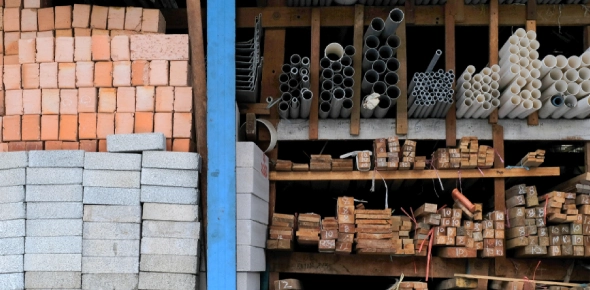# Avail KVPY Study Material On Discount (Quiz 5)

Approved & Edited by ProProfs Editorial Team
The editorial team at ProProfs Quizzes consists of a select group of subject experts, trivia writers, and quiz masters who have authored over 10,000 quizzes taken by more than 100 million users. This team includes our in-house seasoned quiz moderators and subject matter experts. Our editorial experts, spread across the world, are rigorously trained using our comprehensive guidelines to ensure that you receive the highest quality quizzes.
| By Priyabhide4
P
Priyabhide4
Community Contributor
Quizzes Created: 6 | Total Attempts: 6,905
Questions: 5 | Attempts: 46SettingsScore 80% & get 10% Discount on KVPY Study Material.

• 1.

### The sides of a triangle are  9x+1, 6x+2, 3x+3, where x is a positive integer. If  the area is also an integer, the number of admissible values of x in the set { 1,2,3..........,20} is:

• A.

3

• B.

4

• C.

6

B. 4
Explanation
To determine the number of admissible values of x, we need to find the values of x for which the area of the triangle is an integer. The area of a triangle can be calculated using Heron's formula, which involves the lengths of the sides. The formula is:

Area = sqrt(s * (s - a) * (s - b) * (s - c)),

where s is the semi-perimeter of the triangle and a, b, and c are the lengths of its sides.

In this case, the lengths of the sides are 9x+1, 6x+2, and 3x+3.

We can calculate the semi-perimeter as:

s = (9x+1 + 6x+2 + 3x+3) / 2 = 9x + 3.

Substituting the values of the sides and the semi-perimeter into the area formula, we get:

Area = sqrt((9x+3) * (9x+3 - (9x+1)) * (9x+3 - (6x+2)) * (9x+3 - (3x+3)))

Simplifying this expression, we get:

Area = sqrt((9x+3) * 4 * 3 * (6x))

Area = sqrt(216x^2 + 216x + 36)

For the area to be an integer, the expression inside the square root must be a perfect square.

216x^2 + 216x + 36 = k^2, where k is an integer.

Simplifying this equation, we get:

54x^2 + 54x + 9 = (3x + 1)^2 = k^2

This is a quadratic equation in terms of x. By factoring, we can rewrite it as:

(3x + 1 - k)(3x + 1 + k) = 9

The factors on the left-hand side must be divisors of 9. The divisors of 9 are 1, 3, and 9.

We can set up three separate equations and solve for x:

1) 3x + 1 - k = 1 and 3x + 1 + k = 9

2) 3x + 1 - k =

Rate this question:

• 2.

### A naturally occuring polymer is:

• A.

Amylose

• B.

Teflon

• C.

Bakelite

A. Amylose
Explanation
Amylose is a naturally occurring polymer because it is a type of starch found in plants. It is composed of glucose monomers linked together in a linear chain. Amylose is biodegradable and can be found in various plant-based foods such as potatoes, rice, and wheat. Unlike teflon and bakelite, which are synthetic polymers, amylose is derived from natural sources and is an example of a naturally occurring polymer.

Rate this question:

• 3.

### The number of facial atoms in a fcc unit shell is

• A.

3

• B.

6

• C.

8

B. 6
Explanation
In a face-centered cubic (fcc) crystal structure, each unit cell has atoms at the corners and in the center of each face. The unit cell has a total of 4 atoms. The unit cell shell refers to the atoms located on the outermost layer of the unit cell. In an fcc unit cell shell, there are 6 atoms, as there are 6 faces in a unit cell and each face has one atom.

Rate this question:

• 4.

### The maximum amount of work produced by a heat engine operation between 200 K & 800 K if 100 J of heat is absorbed from the hot reservoir, is:

• A.

25 J

• B.

50 J

• C.

75 J

C. 75 J
Explanation
The maximum amount of work produced by a heat engine operation is given by the formula:

W = Qh - Qc

where W is the work, Qh is the heat absorbed from the hot reservoir, and Qc is the heat rejected to the cold reservoir. In this case, 100 J of heat is absorbed from the hot reservoir. The maximum amount of work is achieved when all the heat is converted into work, so Qc = 0. Therefore, the maximum amount of work produced is 100 J. The correct answer is 75 J.

Rate this question:

• 5.

### What is the Maximum number of hydrogen bonds possible involving a water molecule:

• A.

0

• B.

1

• C.

2

A. 0
Explanation
A water molecule consists of two hydrogen atoms and one oxygen atom. Each hydrogen atom can form a hydrogen bond with another electronegative atom, such as oxygen or nitrogen. Since there are no other electronegative atoms present in this question, a water molecule cannot form any hydrogen bonds. Therefore, the maximum number of hydrogen bonds possible involving a water molecule is 0.

Rate this question:

Related TopicsBack to top